# Calculation of financial indexesIn this article, Nithisha CHALLA (ESSEC Business School, Grande Ecole Program – Master in Management, 2021-2023) explains the calculation of financial indexes.

## Introduction

A stock market index keeps tabs on the gains and losses made by a specific selection of stocks or other assets. In other words, the index determines how share prices for various companies have changed. The performance of a market index can be quickly evaluated to ascertain the state of the stock market. It also serves as a template for financial institutions to use when creating index funds and exchange-traded funds (ETFs).

## Definition

What is an index? In financial markets, there are many sectors, segments and business lines, and if you have to statistically measure the performance of these sectors we need a reference which is called an index. Simply, it is a group of securities or financial instruments which represents the performance of a specific segment of the market.

## Calculation

Then the index value has to be calculated with a specific formula. There are different calculation methods for financial indexes: price-weighted index, market-capitalization-weighted index, equal-weighted index and fundamentals-weighted index.

The general formula for a financial index is given by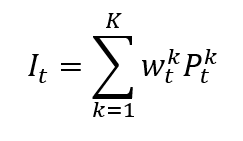where I is the index value, P the asset market price, k a given asset, K the number of assets in the index, wk the weight of asset k in the index, and t is the time of calculation of the index.

Note: the index It at time t is divided by the value of the index at the beginning I0 and multiplied by 100.

### Price-Weighted Index

A price-weighted index is calculated by summing the prices of all the assets in the index and dividing by a divisor equal to the number of assets.

The formula for a price-weighted index is given by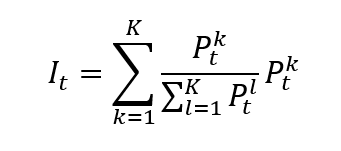where I is the index value, k a given asset, K the number of assets in the index, Pk the market price of asset k, and t the time of calculation of the index.

In a price-weighted index, the weight of asset k is given by formula can be rewritten as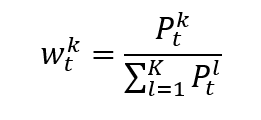which clearly shows that the weight of each asset in the index is its market price divided by the sum of the market prices of all assets.

Note that the divisor, which is equal to the number of shares, is typically adjusted for events such as stock splits and dividends. The divisor is used to ensure that the value of the index remains consistent over time despite changes in the number of outstanding shares. A more general formula may then be: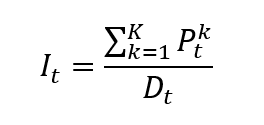where D is the divisor which is adjusted over time to account for events such as stock splits and dividends.

In a price-weighted index, the higher-priced stocks move the index more than the lower-priced stocks.

The most popular price-weighted index in the world is likely the Dow Jones Industrial Average (DJIA). It consists of 30 different stocks in the US market.

### Market-Capitalization-Weighted Index

A market capitalization-weighted index is calculated by multiplying the price of each asset in the index by its number of outstanding shares and summing the resulting values. The weighting of each asset in the index is determined by its market capitalization, so that the largest and most influential companies have the greatest impact on the overall performance of the index.

The formula for a market-capitalization-weighted index is given by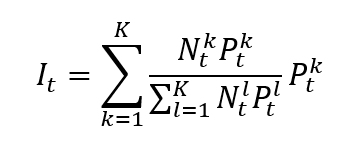where I is the index value, k a given asset, K the number of assets in the index, Pk the market price of asset k, Nk the number of issued shares for asset k, and t the time of calculation of the index.

In a market capitalization-weighted index, the weight of asset k is given by formula can be rewritten as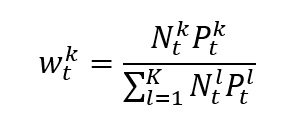which clearly shows that the weight of each asset in the index is its market capitalization of the asset divided by the sum of the market capitalizations of all assets.

Note that the divisor, whose calculation is based on the number of shares, is typically adjusted for events such as stock splits and dividends. The divisor is used to ensure that the value of the index remains consistent over time despite changes in the number of outstanding shares.

In a float-adjusted market-capitalization-weighted index, the market-capitalization weight of each asset is adjusted for its market float. It is also called a free float. Instead of taking into account shares held by insiders, governments, or other entities that might not be available for trading, the weight is adjusted based on the percentage of shares that are actually traded on the open market.

This differs from the market capitalization weighted index as it accounts for the shares outstanding of a company. A float-adjusted market capitalization-weighted index only takes into account shares that are freely available for trading, whereas a market capitalization-weighted index takes into account all outstanding shares, providing a more accurate picture of the performance of the market.

The formula for a float-adjusted market-capitalization-weighted index is given by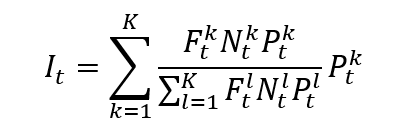where I is the index value, k a given asset, K the number of assets in the index, Pk the market price of asset k, Nk the number of issued shares for asset k, Fk the float factor of asset k, and t the time of calculation of the index.

In a float-adjusted market-capitalization-weighted index, the weight of asset k is given by formula can be rewritten as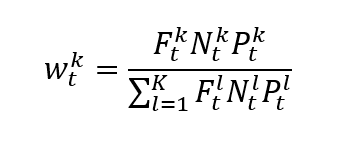### Fundamental-weighted Index

A fundamental-weighted index is calculated based on specific financial metrics, such as revenue or earnings, rather than market capitalization or price. The weightings of each asset in the index are determined by its financial metrics, so that the companies with the strongest financial performance have the greatest impact on the overall performance of the index.

The formula for a fundamental-weighted index is given by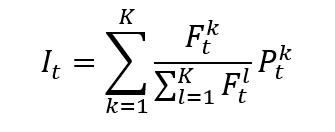where I is the index value, k a given asset, K the number of assets in the index, Pk the market price of asset k, Fk the financial metric of asset k, and t the time of calculation of the index.

In a fundamental-weighted index, the weight of asset k is given by formula can be rewritten as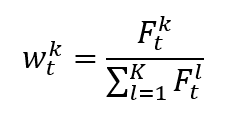which clearly shows that the weight of each asset in the index is the value of the fundamental variable of the asset divided by the sum of the values of the fundamental variable of all assets.

### Equal-weighted Index

An equal-weighted index is calculated by dividing the total value of the index by the number of securities in the index, and then allocating the same weighting to each security. This method gives each security an equal influence on the overall performance of the index, regardless of its market capitalization.

The formula for an equal-weighted index is given by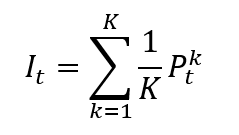In an equal-weighted index, the weight of asset k is given by formula can be rewritten as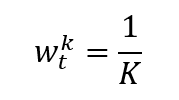Which clearly shows that the weight of each asset in the index, one divided by the number of assets, is constant over time.

## Examples of financial indexes

### The Dow Jones Industrial Average: an equal-weighted index

The Dow Jones Industrial Average, or DJIA (Dow), was the first index, appearing in 1896. The 30 largest and most prosperous American companies make up the Dow. The experts have carefully chosen these businesses to represent a wide range of industries. Companies with higher prices are given more weight in the Dow. Even though it is the most established and performs similarly to the S&P 500, it is occasionally thought to be less indicative of the entire market.

### The S&P 500 index: a market-capitalization-weighted index

S&P 500 – The performance of 500 of the biggest American publicly traded companies is measured. Some people think the S&P 500, which is weighted by market capitalization and has a wider scope, is the best indicator of the American stock market. Because of this, the S&P 500’s average is most significantly impacted by the companies with the highest total market value.

## Why should I be interested in this post?

Learning about the calculation of financial indices is important to understand the behavior of an index. It can assist you in managing risk in your portfolio, understanding the overall performance of various markets, and making wise investment decisions. Financial indices can offer insightful data on how various markets, sectors, and economies are performing. Investors can determine whether their investments are outperforming or underperforming the overall market by comparing the returns to the returns of a relevant financial index.

## Related posts on the SimTrade blog

▶ Nithisha CHALLA Financial indexes

▶ Youssef LOURAOUI Smart Beta strategies: between active and passive allocation

## Useful resources

Weight priced index Indice

Equity Indexes Indice

Security market index Indice

Value weighted index Indice

Evolution of indexes Indice